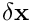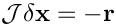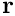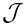Linear solvers

The purpose of this tutorial is to show how to specify different linear solvers for oomph-lib's Newton solver.

# Overview

As discussed in the bottom-up discussion of oomph-lib's data structure, by default oomph-lib's Newton solver, Problem::newton_solve(...) solves the linear systems arising during the Newton iteration with its default linear solver,  SuperLUSolver, a wrapper to Demmel, Eistenstat, Gilbert, Li & Liu's sparse direct solver SuperLU.

oomph-lib provides a large number of alternative linear solvers that may be used instead. All linear solvers within the library are derived from the base class  LinearSolver which contains a single pure virtual function

virtual void solve(Problem* const& problem_pt, DoubleVector& result) = 0;

whose task it is to compute the solution(returned in the vector result) of the linear systemwhereandare the global Jacobian and the residual vector, computed by the Problem pointed to by problem_pt. The  LinearSolver class also defines linear-algebra-type interfaces that allow the solution of linear systems with matrices other than the Problem's Jacobian matrix. However, these methods may not be implemented for all linear solvers.

# List of available linear solvers

oomph-lib's linear solvers can be sub-divided into serial and parallel, and direct and iterative linear solvers. Here is a quick overview of the available linear solvers. If you are viewing this document online, the links take you directly to the solvers' class references which explain any solver-specific member functions.

• Serial solvers:

• Direct solvers:

• SuperLUSolver: oomph-lib's default linear solver, a wrapper to Demmel, Eistenstat, Gilbert, Li & Liu's sparse direct solver SuperLU.

• HSL_MA42: A wrapper to the frontal solver MA42 from the HSL library. This solver is available free-of-charge for UK academics. The source code can be dropped into the oomph-lib distribution; see the instructions in the dummy code

external_src/oomph_hsl/dummy_frontal.f

• DenseLU: A direct solver, based on the LU decomposition of the Jacobian matrix which is stored as a dense matrix. Given that the Jacobian matrices arising from most problems are sparse, this is likely to be a very inefficient solver. It is mainly used by the derived (and even more inefficient!) solver FD_LU.

• FD_LU: Almost certainly the world's most inefficient solver. It computes the Jacobian matrix by finite differencing the global residual vector, without taking any sparsity into account. Mainly used by developers as a last-resort sanity check.

• Iterative solvers:

• oomph-lib provides its own implementations of various standard iterative linear solvers. They are derived from the base class IterativeLinearSolver and are typically templated by the matrix type used store the Jacobian matrix. In most cases you will want to set the template argument MATRIX to CRDoubleMatrix:

• GMRES: A Krylov subspace solver for symmetric and non-symmetric linear systems. The memory usage increases with each iteration but the iteration can be restarted.

• BiCGStab: A Krylov subspace method for symmetric and non-symmetric linear systems. The memory requirement remains constant throughout the iteration.

• CG: The classical conjugate gradient method for symmetric positive definite matrices. The memory requirement remains constant throughout the iteration.
• GS: Gauss-Seidel – a stationary iterative solver.

• oomph-lib also provides wrappers to third-party iterative linear solvers. These tend to provide their own implementations of GMRES, BiCGStab, CG, etc. but are not necessarily derived from oomph-lib's own IterativeLinearSolver base class.

• Parallel solvers:

• Direct solvers:

• When oomph-lib is compiled with MPI support, its default linear solver SuperLUSolver becomes a wrapper to Demmel, Eistenstat, Gilbert, Li & Liu's parallel sparse direct solver SuperLU_DIST. This behaviour can be over-ruled with the member function
SuperLUSolver::set_solver_type(...)
whose argument must specify one of the three options listed in the enumeration SuperLUSolver::Type. This allows the serial solver SuperLU to be used even if oomph-lib is compiled with MPI support.

•  MumpsSolver : is a wrapper to the MUMPS multifrontal solver that is available when oomph-lib is compiled with MPI support and support for MUMPS.
• Iterative solvers:

# How to change the LinearSolver

## Direct solvers

Changing oomph-lib's linear solver is straightforward. For instance, to change the linear solver to oomph-lib's DenseLU solver, simply create an instance of this solver and pass a pointer to it to the Problem. This most easily done in the Problem constructor:

// Change solver to DenseLU
linear_solver_pt()=new DenseLU;

In any subsequent calls to oomph-lib's Newton solver, DenseLU will now be used to solve the linear systems arising during the Newton iteration.

## Iterative linear solvers and preconditioners

The specification of an iterative linear solver is just as easy: For instance, to specify oomph-lib's conjugate gradient solver CG (storing the Jacobian matrix in compressed row format) as the linear solver, add

// Change solver to CG
IterativeLinearSolver* solver_pt=new CG<CRDoubleMatrix>;
linear_solver_pt()=solver_pt;

to the problem constructor. We note that, by default, oomph-lib's IterativeLinearSolvers perform the preconditioning using the trivial "identity preconditioner". Most Krylov subspace solvers perform very poorly without some sort of preconditioning.

Specific preconditioners may be implemented by deriving from the Preconditioner base class, by implementing its two pure virtual functions

/// Apply the preconditioner. Pure virtual generic interface
/// function. This method should apply the preconditioner operator to the
/// vector r and return the vector z.
virtual void preconditioner_solve(const DoubleVector& r,
DoubleVector& z) = 0;

and

/// Setup the preconditioner. Pure virtual generic interface
/// function.
virtual void setup() = 0;

Note that, by default, oomph-lib's IterativeLinearSolvers employ left preconditioning.

oomph-lib provides fully-functional implementations of several general-purpose preconditioners. For instance, the zero-fill-in incomplete LU factorisation preconditioner ILU(0) may be employed by adding the lines

// Specify preconditioner
solver_pt->preconditioner_pt()=new ILUZeroPreconditioner<CRDoubleMatrix>;

to the Problem constructor.

Of particular interest is the availability of an "exact preconditioner"
SuperLUPreconditioner whose use guarantees the convergence of any iterative solver within a single iteration – useful for code development.

## Third-party iterative linear solvers and preconditioners

oomph-lib provides wrappers to various third-party iterative linear solvers and preconditioners. We stress that these solvers are not necessarily implemented as oomph-lib IterativeLinearSolvers since their interfaces for the specification of preconditioners, etc may differ from those employed by oomph-lib. Furthermore, unlike SuperLUSolver these solvers are not distributed as part of oomph-lib so you have to build/install them separately before installing oomph-lib (oomph-lib's build machinery can do this for you if you wish; see installation instructions for details). Once this is done, they may be used like any other linear solver.

### Trilinos

oomph-lib provides wrappers to the iterative linear solvers/preconditioners from the Trilinos Project. The demo code TrilinosSolver_test.cc demonstrates how use various combinations of solvers/preconditioners. Here is a brief overview:

Trilinos solvers

The wrappers to Trilinos' Krylov subspace solvers are implemented as oomph-lib IterativeLinearSolvers, allowing them to be used via the standard interfaces described above. For instance, to use oomph-lib's wrapper to Trilinos' Aztec solver, using Trilinos' ML multilevel preconditioner, set the solvers and preconditioners as usual:

// Create a Trilinos Solver
TrilinosAztecOOSolver* linear_solver_pt = new TrilinosAztecOOSolver;
// Create the Trilinos ML preconditioner
TrilinosMLPreconditioner* preconditioner_pt = new TrilinosMLPreconditioner;
// Set the preconditioner pointer
linear_solver_pt->preconditioner_pt() = preconditioner_pt;
// Set linear solver
problem.linear_solver_pt() = linear_solver_pt;

The actual Krylov subspace solver used by the Trilinos solver is specified by passing an enumerated flag (defined as static member data in the TrilinosAztecOOSolver class) to the solver. For instance, Trilinos' CG, GMRES and BiCGStab solvers are selected with

linear_solver_pt->solver_type() = TrilinosAztecOOSolver::CG;

or

linear_solver_pt->solver_type() = TrilinosAztecOOSolver::GMRES;

or

linear_solver_pt->solver_type() = TrilinosAztecOOSolver::BiCGStab;

respectively.

Trilinos preconditioners

oomph-lib provides wrappers to Trilinos' ML and IFPACK preconditioners that allows them to be used as oomph-lib Preconditioners that may be used with oomph-lib's own IterativeLinearSolvers. Here is an example that shows how to build an instance of oomph-lib's GMRES, preconditioned with its wrapper to Trilinos' IFPACK preconditioner:

// Create oomph-lib linear solver
IterativeLinearSolver* linear_solver_pt=new GMRES<CRDoubleMatrix>;
// Create Trilinos IFPACK preconditioner as oomph-lib Preconditioner
Preconditioner* preconditioner_pt=new TrilinosIFPACKPreconditioner;
// Pass pointer to preconditioner to oomph-lib IterativeLinearSolver
linear_solver_pt->preconditioner_pt()=preconditioner_pt;

### Hypre

oomph-lib provides wrappers to the high-performance linear solvers/preconditioners from the Scalable Linear Solvers Project. The demo code HypreSolver_test.cc demonstrates how use various combinations of solvers/preconditioners. Here is a brief overview:

Hypre solvers

The wrappers to Hypre's Krylov subspace and AMG solvers are implemented as oomph-lib LinearSolvers (not IterativeLinearSolvers!)
so the interfaces for the specification of preconditioners etc. differ from those for oomph-lib's own IterativeLinearSolvers.

The HypreSolver is set like any other LinearSolver:

// Create a new Hypre linear solver
HypreSolver* hypre_linear_solver_pt = new HypreSolver;
// Set the linear solver for problem
problem.linear_solver_pt() = hypre_linear_solver_pt;

The actual solver used by the HypreSolver is specified by passing an enumerated flag (defined as static member data in the HypreSolver class) to the solver. For instance, Hypre's AMG, CG, GMRES and BiCGStab solvers are selected with

hypre_linear_solver_pt->hypre_method() = HypreSolver::BoomerAMG;

or

hypre_linear_solver_pt->hypre_method() = HypreSolver::CG;

or

hypre_linear_solver_pt->hypre_method() = HypreSolver::GMRES;

or

hypre_linear_solver_pt->hypre_method() = HypreSolver::BiCGStab;

respectively.

These Krylov subspace methods may then be preconditioned by Hypre's own (internal) preconditioners, again by specifying the method via an enumerated flag. So, to use no preconditioning, or to precondition with BoomerAMG (and AMG-based preconditioner), Euclid (an ILU preconditioner) or ParaSails (a sparse approximate inverse preconditioner), set:

hypre_linear_solver_pt->internal_preconditioner()=HypreSolver::None;

or

hypre_linear_solver_pt->internal_preconditioner()=HypreSolver::BoomerAMG;

or

hypre_linear_solver_pt->internal_preconditioner()=HypreSolver::Euclid;

or

hypre_linear_solver_pt->internal_preconditioner()=HypreSolver::ParaSails;

respectively.

Hypre preconditioners

oomph-lib provides wrappers to Hypre's preconditioners that allows them to be used as oomph-lib Preconditioners that may be used with oomph-lib's own IterativeLinearSolvers. Here is an example that shows how to build an instance of oomph-lib's BiCGStab and to use the HyprePreconditioner as the preconditioner:

// Build and instance of BiCGStab and pass it to the problem
oomph_linear_solver_pt = new BiCGStab<CRDoubleMatrix>;
problem.linear_solver_pt() = oomph_linear_solver_pt;

Now we build an instance of a HyprePreconditioner

// Create a new Hypre preconditioner
HyprePreconditioner* hypre_preconditioner_pt = new HyprePreconditioner;

and set it as the Preconditioner for oomph-lib's BiCGStab solver:

oomph_linear_solver_pt->preconditioner_pt()=hypre_preconditioner_pt;

The actual preconditioning methodology to be used by the HyprePreconditioner is again selected via enumerated flags, i.e.

hypre_preconditioner_pt->hypre_method() = HyprePreconditioner::BoomerAMG;

or

hypre_preconditioner_pt->hypre_method() = HyprePreconditioner::Euclid;

or

hypre_preconditioner_pt->hypre_method() = HyprePreconditioner::ParaSails;

# Problem-specific preconditioners

In addition to "general-purpose" preconditioners like ILU, oomph-lib provides a number of problem-specific preconditioners which are typically based on the library's block preconditioning framework. Separate documentation is available for these: